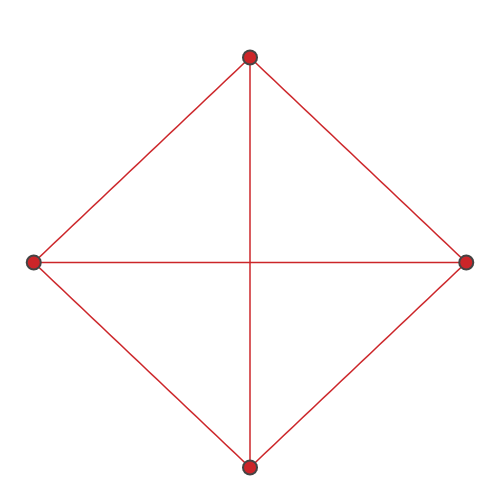sf.apps.plot.subgraph¶

subgraph(s, plot_size=(500, 500))[source]

Creates a plot of the input subgraph.

Subgraphs are plotted using the Kamada-Kawai layout with an aspect ratio of 1:1.

Example usage:

>>> graph = nx.complete_graph(10)
>>> subgraph = graph.subgraph([0, 1, 2, 3])
>>> fig = plot.subgraph(subgraph)
>>> fig.show()Parameters
• s (nx.Graph) – input subgraph

• plot_size (int) – size of the plot in pixels, given as a pair of integers (x_size, y_size)

Returns

figure for subgraph

Return type

Figure